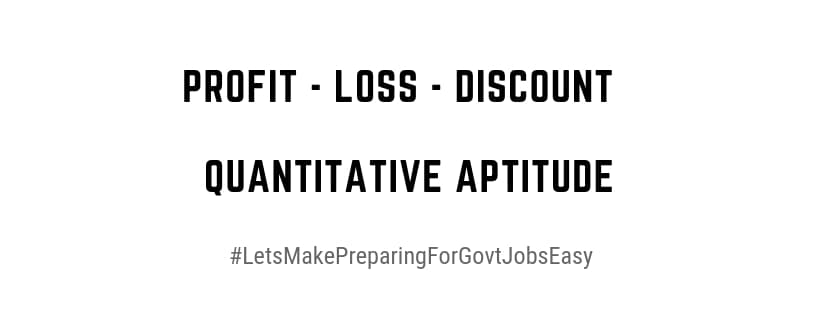Home > Aptitude and Reasoning > PROFIT LOSS DISCOUNTS PROBLEMS WITH SOLUTIONS

# PROFIT LOSS DISCOUNTS PROBLEMS WITH SOLUTIONS# Profit Loss Discounts Problems With Solutions

Quantitative and Aptitude with chapter wise explanations, Profit Loss Discounts Problems With Solutions PDF Download

Profit = Selling price (S. P) – Cost price (C. P)
Loss = Cost price (C. P) – Selling price (S. P)
Profit percentage (P %) = ( Profit/Cost price ) × 100
Loss percentage (L %) = ( Loss /Cost price ) × 100

Selling price (S. P) = (100 + Profit%) /  × Cost price
Selling price (S. P) = (100− Loss%) / × Cost price

NOTE: Profit% and Loss% are always calculated on the Cost price
Discount = Marked Price – Selling Price

Discount % = ( Discount/ Marked Price) × 100
Marked Price = ( 100+ Profit% / 100− Loss%) × Cost Price

The above formula is useful for solving several problems in Profit and Discounts.